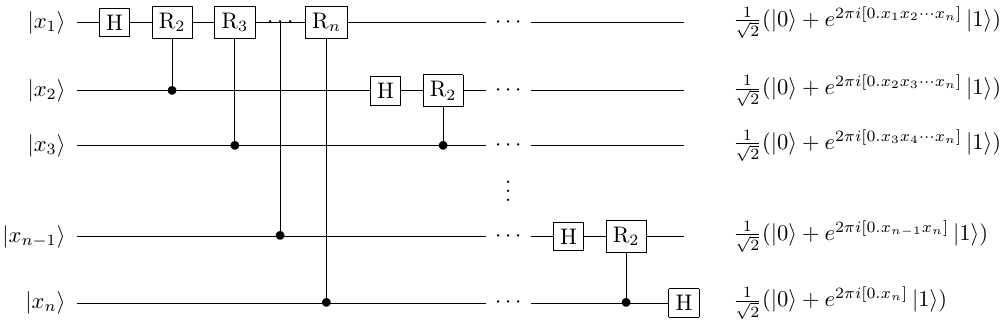# 量子傅里叶变换¶

## 基本定义¶

\begin{split}\begin{aligned} y_k\rightarrow\frac{1}{\sqrt N}\mathrm{\Sigma}_{j=0}^{N-1}x_j \ e^{\frac{2\pi\ i}{N}jk},\\ \left|x\right\rangle\rightarrow \ \frac{1}{2^\frac{n}{2}}\Sigma_{k=0}^{2^n-1}e^{\frac{2\pi i}{2^n} \ xk}\left|k\right\rangle. \end{aligned}\end{split}

## 量子线路构造¶

### QFT的求和形式与张量积形式¶

\begin{split}\begin{aligned} & QFT(\left|x\right\rangle)=\frac{1}{2^\frac{n}{2}}\mathrm{\Sigma}_{k=0}^{2^n-1}e^\frac{2\pi ixk}{2^n} \ \left|k\right\rangle=\frac{1}{2^\frac{n}{2}}\mathrm{\Sigma}_{k_1=0}^1\cdots\mathrm{\Sigma}_{k_n=0}^1 \ e^{2\pi ixk\left(\mathrm{\Sigma}_{l=1}^nk_l2^{-l}\right)}\left|k_1\cdots k_n\right\rangle \\ & =\frac{1}{2^\frac{n}{2}}\mathrm{\Sigma}_{k_1=0}^1\cdots\mathrm{\Sigma}_{k_n=0}^1\otimes_{l=1}^n e^{2\pi ix k_l2^{-l}} \left|k_l\right\rangle=\frac{1}{2^\frac{n}{2}}\otimes_{l=1}^n(\left|0\right\rangle+e^{2\pi ix2^{-l}} \ \left|1\right\rangle). \end{aligned}\end{split}

### 二进制展开与量子态制备¶

\begin{aligned} x/2^m \approx \left[x_1\cdots x_m\right]/2^m=\left[0.x_1\cdots x_m\right]=\Sigma_{k=1}^mx_k2^{-k}, \end{aligned}

\begin{aligned} 2\pi ix2^{-l}=2\pi i\left[x_1\cdots x_n\right]2^{-l}=2\pi i\left[0.x_{n-l}\cdots x_n\right]. \end{aligned}

\begin{split}\begin{aligned} & \frac{1}{\sqrt{2}}(\left|0\right\rangle + e^{2\pi i [0.x_{n-1} x_n]}\left|1\right\rangle) = \ \frac{1}{\sqrt{2}}(\left|0\right\rangle + e^{2\pi i [0.x_{n-1}]} e^{2\pi i [0.0 x_n]} \left|1\right\rangle),\\ & R_m \left|0\right\rangle = \left|0\right\rangle, R_m \left|1\right\rangle = e^{2\pi i \frac{1}{2^m}}\left|1\right\rangle. \end{aligned}\end{split}

\begin{aligned} (C-R)_{j-k+1} \frac{1}{\sqrt{2}}(\left|0\right\rangle + e^{2\pi i [0.x_{n-j}]} \left|1\right\rangle)\left|x_{n-k}\right\rangle = \frac{1}{\sqrt{2}}( \left|0\right\rangle + e^{2\pi i [0.x_{n-j}0\cdots 0x_{n-k}]}\left|1\right\rangle. \end{aligned}

QFT的量子线路图如下所示## 代码实现¶

QFT(qlist)


#!/usr/bin/env python

import pyqpanda as pq
from numpy import pi

if __name__ == "__main__":

machine = pq.init_quantum_machine(pq.QMachineType.CPU)
qvec = machine.qAlloc_many(3)
prog = pq.create_empty_qprog()

# 构建量子程序
prog.insert(pq.QFT(qvec))

# 对量子程序进行概率测量
result = pq.prob_run_dict(prog, qvec, -1)
pq.destroy_quantum_machine(machine)

# 打印测量结果
for key in result:
print(key+":"+str(result[key]))


000, 0.125
001, 0.125
010, 0.125
011, 0.125
100, 0.125
101, 0.125
110, 0.125
111, 0.125